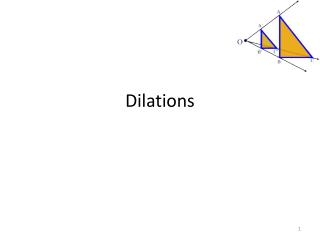Download PresentationDilations

# Dilations - PowerPoint PPT Presentation

Dilations. Why dilate?. Some photographers prefer traditional cameras and film to produce negatives. From these negatives, photographers can create scaled drawings. Why dilate?. Scientists need to understand dilations when examining organisms under a microscope. Why dilate?.I am the owner, or an agent authorized to act on behalf of the owner, of the copyrighted work described.
Download Presentation## Dilations

Download Policy: Content on the Website is provided to you AS IS for your information and personal use and may not be sold / licensed / shared on other websites without getting consent from its author.While downloading, if for some reason you are not able to download a presentation, the publisher may have deleted the file from their server.

- - - - - - - - - - - - - - - - - - - - - - - - - - E N D - - - - - - - - - - - - - - - - - - - - - - - - - -
Presentation Transcript
1. Dilations

2. Why dilate? • Some photographers prefer traditional cameras and film to produce negatives. From these negatives, photographers can create scaled drawings.

3. Why dilate? • Scientists need to understand dilations when examining organisms under a microscope.

4. Why dilate? • Crime labs investigate DNA, hair samples, and fingerprints to solve cases.

5. Why dilate? • Architects design buildings and structures on the computer and create blueprints from which builders and engineers construct.

6. Key Concepts • The image and pre-image of a figure are similar • A dilation with center C and scale factor k • This is a type of transformation, not rigid, but non-rigid • A dilation is a reduction if 0<k<1 • A dilation is an enlargement if k>1

7. Similar Figures (Not exactly the same, but pretty close!)

8. Congruent Figures In order to be congruent, two figures must be the exact same size and same shape (isometry)

9. Similar Figures Similar figures must be the same shape, but their sizes may be different. Their sides must be proportional.

10. 9 cm W Z 3 cm A D 2 cm 2 cm 6 cm 6 cm B C 3 cm Y X 9 cm In the rectangles above, one proportion is = , or = . AB WX AD WZ 2 6 3 9 Similar Figures If you cannot use corresponding side lengths to write a proportion, or if corresponding angles are not congruent, then the figures are not similar.

11. SIZES Although the size of the two shapes are different, the sizes of the two shapes must differ by a factor. 4 2 3 3 6 6 1 2 In this case, the factor is 2.

12. Enlargements When you have a photograph enlarged, you make a similar photograph. X 3

13. Reductions A photograph can also be shrunk to produce a slide. 4

14. Similarity is used to answer real life questions. Suppose that you wanted to find the height of this tree. Unfortunately all that you have is a tape measure, and you are too short to reach the top of the tree.

15. Then, measure the length of your shadow. 10 feet 2 feet

16. If you know how tall you are, then you can determine how tall the tree is. 6 ft 10 feet 2 feet

17. The tree must be 30 ft tall. Boy, that’s a tall tree! 6 ft 10 feet 2 feet

18. Determine the length of the unknown side. 15 12 ? 4 3 9

19. Sometimes the factor between 2 figures is not obvious and some calculations are necessary. 15 12 8 10 18 12 ? =

20. When changing the size of a figure, will the angles of the figure also change? ? 40 70 ? ? 70

21. Nope! Remember, the sum of all 3 angles in a triangle MUST add to 180 degrees.If the size of theangles were increased,the sum would exceed180degrees. 40 40 70 70 70 70

22. We can verify this fact by placing the smaller triangle inside the larger triangle. 40 40 70 70 70 70

23. The 40 degree angles are congruent. 40 70 70 70 70

24. The 70 degree angles are congruent. 40 40 70 70 70 70 70

25. The other 70 degree angles are congruent. 4 40 70 70 70 70 70

26. Find the length of the missing side. 50 30 ? 6 40 8

27. This looks messy. Let’s translate the two triangles. 50 30 ? 6 40 8

28. Now “things” are easier to see. 50 30 ? 6 40 8

29. The common factor between these triangles is 5. 50 30 ? 6 40 So the length of the missing side is…? 8

30. Similar Figures • Corresponding sides have lengths that are proportional. • Corresponding angles are congruent.

31. Dilations in the Coordinate Plane This is the rule only when the dilation is centered at the origin. We will dilate figures that are not centered at the origin as well.

32. Guided Practice Triangle DEF has vertices D(-1,-2), E(-2,2), and F(1,1). Find the coordinates of triangle D’E’F’, after a dilation of -2.

33. Real-World Application

34. Homework: • Finish the back of the class work sheet • Tomorrow we will be incorporating the translations we learned from the last unit, so PLEASE REVIEW THEM 